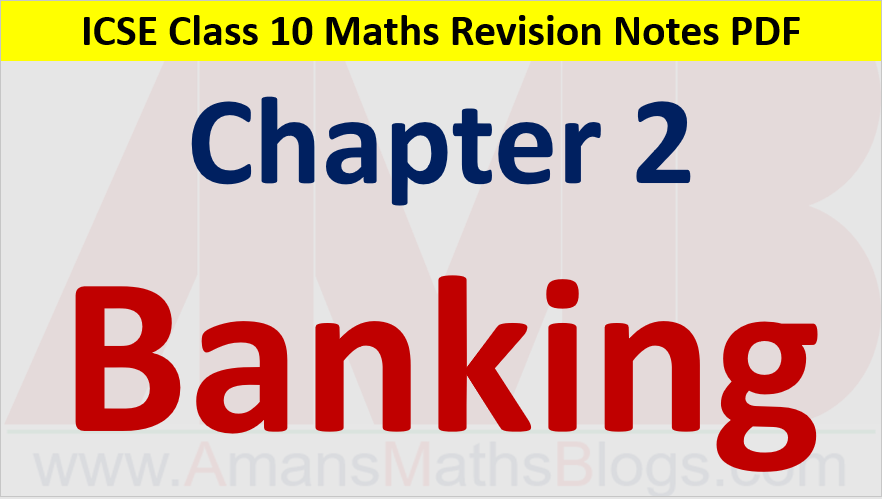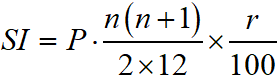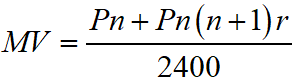Friday, October 22, 2021
Home > ICSE Solutions for Class 10 Maths > Banking Class 10 ICSE Maths Revision Notes Chapter 2 PDF

# Banking Class 10 ICSE Maths Revision Notes Chapter 2 PDFHi students, Welcome to Amans Maths Blogs (AMB). In this article, you will get Banking ICSE Class 10 Maths Revision Notes & Solutions Chapter 2 PDF.

## What is Fixed Deposits (FD)?

In fixed deposit, a depositor deposits a fixed amount for a fixed period of time. At the expiry of this period, the depositor gets the amount deposited by him together with an interest. For fixed deposits, the minimum duration is 7 days and for recurring deposit, the minimum duration is six months. The maximum duration for both schemes FD and RD is 10 years

## What is Recurring Deposits (RD)?

In this scheme, a depositor deposits a fixed amount every month for a specified months. At the expiry of this period, the depositor gets the amount deposited by him together with an interest, completed quarterly at a fixed rate.

## What is Fixed Deposits (FD)?

In fixed deposit, a depositor deposits a fixed amount for a fixed period of time. At the expiry of this period, the depositor gets the amount deposited by him together with an interest. For fixed deposits, the minimum duration is 7 days and for recurring deposit, the minimum duration is six months. The maximum duration for both schemes FD and RD is 10 years.

## What is difference FD and RD?

In RD, an investment is made at a fixed period, whereas in FD, the investment is one-time. RD works well for people who wish to invest minimal amount monthly or at any fixed period. The investment in FD is a higher lumpsum. When returns in FD or RD are compared, then FD seems to give higher returns.

## What is Recurring Deposits Formula (RD Formula)?

If Rs. P is the money deposited per month, n, the number of months for which this money is deposited and r% p.a. the simple interest, then the equivalent principle for 12 months is Rs. Pn(n + 1)/2, time = 1 month, r rate of interest = r/12% per month and hence the simple interest isThus, the maturity value is MV = Pn + SI, orAMBiPi
error: Content is protected !!